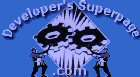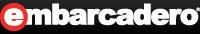Articles Members Online:
 -Article/Tip Search -News Group-Delphi/Pascal -CBuilder/C++ -C#Builder/C# -JBuilder/Java -Kylix Member Area -Home -Account Center -Top 10 NEW!! -Submit Article/Tip -Forums Upgraded!! -My Articles -Edit Information -Login/Logout -Become a Member -Why sign up! -Newsletter -Chat Online! -Indexes NEW!! Employment -Build your resume -Find a job -Post a job -Resume Search Contacts -Contacts -Feedbacks -Link to us -Privacy/Disclaimer
 EmbarcaderoEmbarcadero Community JEDI Links
 22-Feb-04
 Category Multimedia Language Delphi 2.x Views 141 User Rating No Votes # Votes 0 Replies 0
 Publisher: DSP, Administrator Reference URL: DKB
 ``` Author: Eber Irigoyen In this article I'll show you how to create 3D shapes, which is just an extension of the last article, also you'll see how to rotate around the same shape axis or rotate around other object (like the solar system) Answer: Ok, this is the 3rd of a series of articles on OpenGL, so far we have seen: OpenGL I: Hello World, Setup a Delphi OpenGL Application OpenGL II: Moving and rotating 2D shapes Now we're gonna expand on the last article to create 3D shapes, rotate them around their own axis and rotating objects around some other object (like a solar system) To accomplish such thing we're still going to use the same basic OpenGL instructions but because this is graphics, one line in the wrong place makes a huge difference As I stated in my last article, OpenGL just follows instructions like "move forward", "turn left X degrees", etc, so just with that you know is not the same: "move forward 10 units", "turn left 90 degrees" than: "turn left 90 degrees", "move forward 10 units" simple, right?? well, just those simple rules will make a difference when you want to rotate an object around it's own axis or around other object axis Rotating in the object own axis would be something like: "move to 0, 0, 0", "move forward 10 units", "turn left X degrees", "draw a shape", "inc(X, 1.0)" very simple, we move to a fixed position (10 units forward) turn X degrees, X gets incremented later, so that makes the shape rotate around its own axis and then we draw the shape, and increment the angle In Delphi would be something like: 1 glTranslatef(-0.5, 0.0, -15.0); // Move 0.5 Units Left And 15.0 units Into 2 The Screen 3 glRotatef(rquad, 0.0, 1.0, 1.0); // Rotate The Quad On The Y and Z axis ( NEW ) 4 glBegin(GL_QUADS); // We are going to draw a cube, so let's use QUADS 5 glColor3f(0.0, 1.0, 0.0); 6 glVertex3f(0.5, 0.5, -0.5); // Top Left 7 glVertex3f(-0.5, 0.5, -0.5); // Top Right 8 {. 9 . 10 .} 11 glEnd(); 12 13 rquad := rquad + 1.0; Now to rotate around that object we just drew, we would have to do the following: "cancel previous rotation", "turn left X degrees", "move 3 units into screen", "turn right Y degrees", "draw shape" The first important thing to notice here is that we didn't use "move 0, 0, 0", which means we're going to keep drawing from the position where we drew the last shape The second thing is cancelling the previous rotation, why is that? Because if you don't, when you move forward X units, is going to move into whatever direction the last angle was, that might be what you want, but if you want your shape to rotate in a different axis, then you have to cancel the rotation, and then rotate to where you want and then move... confusing? luckily you'll get to play with the source code and see what happens when you cancel and when you don't. The third thing is rotating to the angle that you want (this actually makes the rotation around the first object posible) Move 3 units into screen, This to separate the new shape from the last, if we don't move to any direction, we will draw this shape in the same place!, maybe rotated to a different angle but in the same coordinates Finally we do a last rotation around a new variable angle and draw the shape. This last rotation will make the new object rotate around it's own axis just like the solar system, the earth rotates around the sun, and then also rotates around it's own axis, makes sense? I hope so let's look at the Delphi code: 14 //Notice we don't use glLoadIdentity here, which means we are at whatever 15 position we drew the quad 16 glRotatef(-rquad, 0.0, 1.0, 1.0); // Cancel the previous rotation!!! 17 glRotatef(rtri, 0.0, 1.0, 0.0); // Rotate The Triangle On The Y axis (around the 18 quad) 19 glTranslatef(0.0, 0.0, 3.0); // Move 3.0 Units Into The screen 20 glRotatef(rtri, 0.0, 0.0, 1.0); 21 // Let's rotate the pyramid, while we rotate around the quad 22 glBegin(GL_TRIANGLES); // We're gonna draw a pyramid, so let's use TRIANGLES 23 glColor3f(1.0, 0.0, 0.0); // Red 24 glVertex3f(0.0, 0.5, 0.0); // Top Of Triangle (Front) 25 {. 26 . 27 .} 28 glEnd(); 29 30 rtri := rtri + 2.0; 31 32 //ok, that's it on explanations, here's the source code for the drawing procedure I 33 added a polygon to the scene just to show you how to create them Also check the 34 coloring mix on the pyramid 35 36 function DrawGLScene(): Bool; { All Rendering Done Here } 37 begin 38 glClear(GL_COLOR_BUFFER_BIT or GL_DEPTH_BUFFER_BIT); //Clear Screen and Depth 39 Buffer 40 glLoadIdentity(); //Reset The View 41 42 glTranslatef(-0.5, 0.0, -15.0); // Move 0.5 Units Left And 15.0 units Into The 43 Screen 44 glRotatef(rquad, 0.0, 1.0, 1.0); // Rotate The Quad On The Y and Z axis ( NEW ) 45 glBegin(GL_QUADS); // We are going to draw a cube, so let's use QUADS 46 glColor3f(0.0, 1.0, 0.0); 47 glVertex3f(0.5, 0.5, -0.5); // Top Left 48 glVertex3f(-0.5, 0.5, -0.5); // Top Right 49 glVertex3f(-0.5, 0.5, 0.5); // Bottom Right 50 glVertex3f(0.5, 0.5, 0.5); // Bottom Left 51 52 glColor3f(1.0, 0.5, 0.0); 53 glVertex3f(0.5, -0.5, -0.5); // Top Left 54 glVertex3f(-0.5, -0.5, -0.5); // Top Right 55 glVertex3f(-0.5, -0.5, 0.5); // Bottom Right 56 glVertex3f(0.5, -0.5, 0.5); // Bottom Left 57 58 glColor3f(1.0, 0.0, 0.0); 59 glVertex3f(0.5, 0.5, 0.5); // Top Left 60 glVertex3f(-0.5, 0.5, 0.5); // Top Right 61 glVertex3f(-0.5, -0.5, 0.5); // Bottom Right 62 glVertex3f(0.5, -0.5, 0.5); // Bottom Left 63 64 glColor3f(1.0, 1.0, 0.0); 65 glVertex3f(0.5, -0.5, -0.5); // Top Left 66 glVertex3f(-0.5, -0.5, -0.5); // Top Right 67 glVertex3f(-0.5, 0.5, -0.5); // Bottom Right 68 glVertex3f(0.5, 0.5, -0.5); // Bottom Left 69 70 glColor3f(0.0, 0.0, 1.0); 71 glVertex3f(-0.5, 0.5, 0.5); // Top Left 72 glVertex3f(-0.5, 0.5, -0.5); // Top Right 73 glVertex3f(-0.5, -0.5, -0.5); // Bottom Right 74 glVertex3f(-0.5, -0.5, 0.5); // Bottom Left 75 76 glColor3f(1.0, 0.0, 1.0); 77 glVertex3f(0.5, 0.5, -0.5); // Top Left 78 glVertex3f(0.5, 0.5, 0.5); // Top Right 79 glVertex3f(0.5, -0.5, 0.5); // Bottom Right 80 glVertex3f(0.5, -0.5, -0.5); // Bottom Left 81 glEnd(); 82 83 //Notice we don't use glLoadIdentity here, which means we are at whatever 84 position we drew the quad 85 glRotatef(-rquad, 0.0, 1.0, 1.0); // Cancel the previous rotation!!! 86 glRotatef(rtri, 0.0, 1.0, 0.0); 87 // Rotate The Triangle On The Y axis (around the quad) 88 glTranslatef(0.0, 0.0, 3.0); // Move 3.0 Units Into The screen 89 glRotatef(rtri, 0.0, 0.0, 1.0); 90 // Let's rotate the pyramid, while we rotate around the quad 91 glBegin(GL_TRIANGLES); // We're gonna draw a pyramid, so let's use TRIANGLES 92 glColor3f(1.0, 0.0, 0.0); // Red 93 glVertex3f(0.0, 0.5, 0.0); // Top Of Triangle (Front) 94 glColor3f(0.0, 1.0, 0.0); // Green 95 glVertex3f(-0.5, -0.5, 0.5); // Left Of Triangle (Front) 96 glColor3f(0.0, 0.0, 1.0); // Blue 97 glVertex3f(0.5, -0.5, 0.5); // Right Of Triangle (Front) 98 99 glColor3f(1.0, 0.0, 0.0); // Red 100 glVertex3f(0.0, 0.5, 0.0); // Top Of Triangle (Right) 101 glColor3f(0.0, 0.0, 1.0); // Blue 102 glVertex3f(0.5, -0.5, 0.5); // Left Of Triangle (Right) 103 glColor3f(0.0, 1.0, 0.0); // Green 104 glVertex3f(0.5, -0.5, -0.5); // Right Of Triangle (Right) 105 106 glColor3f(1.0, 0.0, 0.0); // Red 107 glVertex3f(0.0, 0.5, 0.0); // Top Of Triangle (Back) 108 glColor3f(0.0, 1.0, 0.0); // Green 109 glVertex3f(0.5, -0.5, -0.5); // Left Of Triangle (Back) 110 glColor3f(0.0, 0.0, 1.0); // Blue 111 glVertex3f(-0.5, -0.5, -0.5); // Right Of Triangle (Back) 112 113 glColor3f(1.0, 0.0, 0.0); // Red 114 glVertex3f(0.0, 0.5, 0.0); // Top Of Triangle (Left) 115 glColor3f(0.0, 0.0, 1.0); // Blue 116 glVertex3f(-0.5, -0.5, -0.5); // Left Of Triangle (Left) 117 glColor3f(0.0, 1.0, 0.0); // Green 118 glVertex3f(-0.5, -0.5, 0.5); // Right Of Triangle (Left) 119 glEnd(); 120 121 glLoadIdentity(); // Move to (0, 0, 0) 122 glTranslatef(1.5, 0.0, -6.0); // Move 1.5 Right and -6.0 intro screen 123 glRotatef(rpol, 0.0, 0.0, 1.0); // rotate on Z axis 124 glColor3f(0.0, 0.0, 1.0); // Add some color 125 glBegin(GL_POLYGON); // Draw A Polygon (I can put many points in here) 126 glVertex3f(-0.5, 0.5, 0.0); // Top Left 127 glVertex3f(0.0, 0.75, 0.0); // Upper point 128 glVertex3f(0.5, 0.5, 0.0); // Top Right 129 glVertex3f(0.5, -0.5, 0.0); // Bottom Right 130 glVertex3f(0.0, -0.75, 0.0); // Lower point 131 glVertex3f(-0.5, -0.5, 0.0); // Bottom Left 132 glEnd(); 133 134 rtri := rtri + 2.0; 135 rquad := rquad + 1.0; 136 rpol := rpol + 1.0; 137 138 Result := True 139 end; That's it, I hope it wasn't too confusing, play with the code, see what happens when you comment this or that line. ```